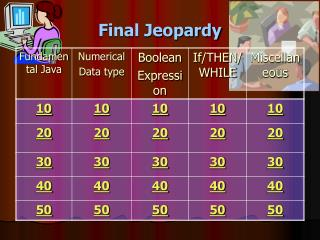DownloadDownload PresentationFinal Jeopardy

# Final Jeopardy

Download Presentation## Final Jeopardy

- - - - - - - - - - - - - - - - - - - - - - - - - - - E N D - - - - - - - - - - - - - - - - - - - - - - - - - - -
##### Presentation Transcript

1. Final Jeopardy

2. Java fundamental for 10 Q: How to compile and run a Java program? OL 10 A

3. Java fundamental– 10 points A: What is Javac and Java? Table

4. Java fundamental for 20 Q: A Java program contains one or more of this? OL 20 A

5. Java fundamental for 20 A: What is CLASS? Table

6. Java fundamental for 30 Q: This is needed to run the same Java program on different Operating Systems without having to recompile? OL 30 A

7. Java Fundamental for 30 A: What is Java Virtual Machine (JVM) ? Table

8. Java fundamental for 40 Q: The name of this tree has been used as another name for Java ? OL 40 A

9. Java fundamental for 40 A: What is “OAK”? Table

10. Java fundamental for 50 Q: This used to be the best selling point for Java (hint: refer to its portability with 4 words) OL 50 A

11. Java fundamental for 50 A: What is “Write ONCE Run EVERYWHERE”? Table

12. Numerical Data type 10 Q: Data types used to represent integer values? CF 10 A

13. Numerical Data for 10 A: What are byte, short, int, long? Table

14. Numerical Data for 20 Q: Data types to represent real values. CF 20 A

15. Numerical data types for 20 A: What are float and double? Table

16. Numerical data type for 30 Q: These symbols are allowed in a valid identifier? CR 30 A

17. Numerical data type for 30 A: What are \$ and _? Table

18. Numerical Data type for 40 Q: Suppose we have: int x, y; x = 40; y = x / 6 What is the value of y? CF 40 A

19. Numerical Data for 40 A: What is 6? Table

20. Numerical data for 50 Q: The values of this can not be changed in the entire program? CF 50 A

21. Numerical data for 50 A: What is constant? Table

22. Boolean expression for 10 Q: Boolean expressions are evaluated to one of these values? IS 10 A

23. Boolean expression for 10 A: What is true or false? Table

24. Boolean expression for 20 Q: These are boolean operators in Java? IS 20 A

25. Boolean Expression for 20 A: What are AND, OR, NOT? Table

26. Boolean expression for 30 Q: Which are the six relational operators used in boolean expressions? • < • >= • <= • > • == • && • != • ! IS 30A

27. Boolean expression for 30 A: What are A,B,C,D,E,and G? Table

28. Boolean Expression for 40 Q: Evaluate this boolean expression: (1<2 || 4 <=3) IF 40 A

29. Boolean expression for 40 A: What is true? Table

30. Boolean expression for 50 Q: Evaluate this expression: (60%3 == 0) && (50%9 != 0) IF 50 A

31. Boolean expression for 50 A: What is true? Table

32. If/then/while/for for 10 Q: What is the output from the following code?. int number = 45; if (number != 45) { System.out.println(“Hello”); } else { System.out.println(“Bye”); } A. Hello B. Bye T10 A

33. If/Then/While for 10 A: What is B? Table

34. If/Then/While for 20 Q: What is the result of this switch statement: int number = 45; switch (number) { case 35: System.out.println(“Good”); case 45: System.out.println(“Fair”); case 55: System.out.println(“None”); } A. Good B. Fair C. None T20 A

35. If/Then/While for 20 A: What is Fair? Table

36. If/Then/While for 30 Q: What is this code doing? if (num1 * num2 * num3 < 0) { System.out.println(“This is good”); } else { System.out.println(“This is bad”); } T30 A

37. If/Then/While for 30 A: What is “if products of these three numbers (num1,num2,num3) are negative, print out “This is good”. Otherwise, print out “This is bad” ? Table

38. If/Then/While for 40 Q: These symbols are always found in an if statement T40 A

39. If/Then/While for 40 A: What are ( and )? Table

40. It/Then/While for 50 Q: The statements in the body of this loop must be executed at least ONCE T50 A

41. If/Then/While for 50 A: What is DO-WHILE loop? Table

42. Miscellaneous for 10 Q: Switch only support these data types ?. DM 10 A

43. Miscellaneous for 10 A: What are char and integer (byte, short, int)? Table

44. Miscellaneous for 20 Q: Operand is converted from a lower to a higher precision DM 20 A

45. Miscellaneous for 20 A: What is implicit casting? Table

46. Miscellaneous for 30 Q: How can we say 23 in Java. DM 30 A

47. Miscellaneous for 30 A: What is Math.pow(2,3) ? Table

48. Miscellaneous for 40 Q: JOptionPane.showInputDialog gets input from a user and return this data type DM 40 A

49. Miscellaneous for 40 A: What is String? Table

50. Miscellaneous for 50 Q: The value of this component can be changed in a Java program DM 50 A